Heart as pump

Heart pumps out 5.17 liters of blood in 1 minute. How many liters of blood pumped per hour and how much per day?

Result

h =  310.2 l
d =  7444.8 l

Solution:Leave us a comment of this math problem and its solution (i.e. if it is still somewhat unclear...):Be the first to comment!To solve this verbal math problem are needed these knowledge from mathematics:

Do you know the volume and unit volume, and want to convert volume units?

Next similar math problems:

1. Pump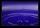From the pump in the yard dripping water. Every drop is 1 ml. How many drops will be necessary before filling a bucket with a volume of 10 l? Calculate how long it will fulfill the bucket when drops fall each second.
2. Infants water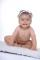From the tank, which has 10 hectoliters of water for infants, went 580 liters at mornening and 340 liters in the afternoon. How many liters left in the tank?
3. Juice box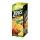In the box is 0.3 liters of juice. How many liters of juice contains 3 these boxes?
4. The shopThe shop has 3 hectoliters of water. How many liter bottles is it?
5. Hr to minSue biked to school in 5/12 of an hour. How many minutes did it take her to ride to school?
6. Tank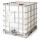How many minutes does it take to fill the tank to 25 cubic meters of water filled 150hl per hour?
7. HoursThe lesson lasts 45 minutes. For the week, students have 18 lesson hours. How many are the actual hours?
8. WorkersThree factory operators produced 480 units in 50 minutes. How many hours worked? I'm trying to prove or disapprove the idea that the company tell me that it's 2.5 hours. So what is right? Thank you, Petra
9. If youIf you travel to work 22 days and it takes 29.2 minutes, how many minutes will it take to travel to work and back?
10. Water tankIn an empty fire tank, 2150 hl of water jetted in 5 hours. How many hectoliters of water was jetted every hour? How many hectoliters of water was in the tank after three hours?
11. Hours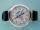How many hours is 9 days?
12. Clock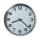How many hours are 15 days?
13. Time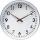16.2 days ..... how many hours is it?
14. Minutes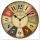Determine the difference in minutes: T1 = 2 3/20 h T2 = 2.3 h
15. Seeds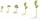Of the 72 wet seeds 7/8 germinated. How many seeds germinate?
16. ProductResult of the product of the numbers 1, 2, 3, 1, 2, 0 is:
17. Addition of Roman numbersAdded together and write as decimal number: LXVII + MLXIV